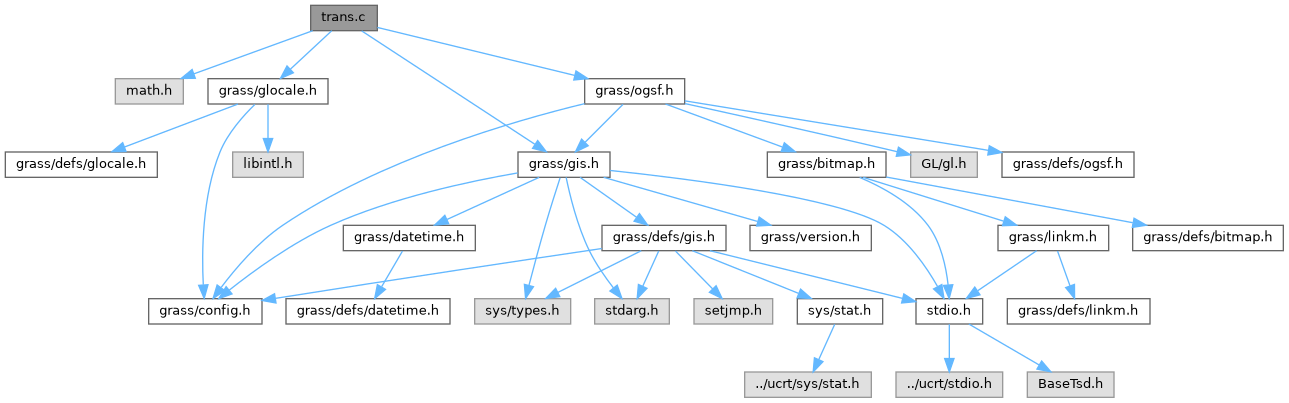GRASS GIS 8 Programmer's Manual  8.2.2dev(2023)-3d37ee165c
trans.c File Reference

OGSF library - matrix transformation (higher level functions) More...

`#include <math.h>`
`#include <grass/gis.h>`
`#include <grass/glocale.h>`
`#include <grass/ogsf.h>`
Include dependency graph for trans.c:Go to the source code of this file.

## Macros

#define MAX_STACK   20

#define NPI   M_PI

## Functions

void P_scale (float x, float y, float z)

void P_transform (int num_vert, float(*in), float(*out))
Transform array of vectors using current T matrix. More...

int P_pushmatrix (void)
Push current transformation matrix onto matrix stack. More...

int P_popmatrix (void)
Pop top of matrix stack, placing it into the current transformation matrix. More...

void P_rot (float angle, char axis)
Rotate matrix. More...

## Detailed Description

OGSF library - matrix transformation (higher level functions)

GRASS OpenGL gsurf OGSF Library

NOTE: This file should be REMOVED and any calls to the functions in this file should be replaced with appropriate OpenGL calls.

This routine should be available in GL!

Arguments are same as GL counterparts

I threw this code together in January at the beginning of this class. I was still learning about GL at the time. There are many places where the code could be improved.

(C) 1999-2008 by the GRASS Development Team

This program is free software under the GNU General Public License (>=v2). Read the file COPYING that comes with GRASS for details.

Definition in file trans.c.

## ◆ MAX_STACK

 #define MAX_STACK   20

Definition at line 37 of file trans.c.

Referenced by P_pushmatrix().

## ◆ NPI

 #define NPI   M_PI

Definition at line 51 of file trans.c.

## ◆ P_popmatrix()

 int P_popmatrix ( void )

Pop top of matrix stack, placing it into the current transformation matrix.

Returns
-1 on failure
0 on success

Definition at line 197 of file trans.c.

References _, and G_warning().

## ◆ P_pushmatrix()

 int P_pushmatrix ( void )

Push current transformation matrix onto matrix stack.

Definition at line 177 of file trans.c.

References _, G_warning(), and MAX_STACK.

Referenced by gsd_update_cpnorm(), and P_scale().

## ◆ P_rot()

 void P_rot ( float angle, char axis )

Rotate matrix.

Parameters
 angle angle value axis ('x, 'y', 'z')

Definition at line 217 of file trans.c.

Referenced by gsd_update_cpnorm().

## ◆ P_scale()

 void P_scale ( float x, float y, float z )

Parameters
 x,y,z

Definition at line 75 of file trans.c.

References P_pushmatrix(), and x.

## ◆ P_transform()

 void P_transform ( int num_vert, float(*) in, float(*) out )

Transform array of vectors using current T matrix.

Multiply 'in' matrix (homogenous coordinate generally) by the current transformation matrix, placing the result in 'out'

[in][trans_mat] => [out]

Parameters
 num_vert in out

Definition at line 117 of file trans.c.